# Videos

BioForce Analytics Lab Videos

## Videos - BundlesBundles are coupon codes which can be used for cart discounts at checkout. Codes can not be combined with other discounts or coupon codes in a single order. You may use coupon codes more than once; however, you will need to place separate orders for each coupon you wish to use.

## Videos - Bundles - Basic BundleLabs 1-5 & 11
One tier
One setup

With this bundle you get everything you need to run 6 different labs. For each lab you get the lab video for one setup at one tier (level), the editable labsheet and the teacher guide. If you choose a tier 3 bundle then you will also get the data files.

## Videos - Bundles - Lab Bundles1 lab
All tiers
All setups

With this bundle you get all of the resources for a single lab. That includes all of the lab videos for each setup at each tier (level), the editable lab sheet and teacher guides.

## Videos - Lab 1 Inclined Plane KinematicsIn the lab students learn how to use kinematics equations to determine the acceleration of a mass along an inclined plane.

## Videos - Lab 1 Inclined Plane Kinematics - Lab 1: Tier 1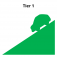In the lab students learn how to use kinematics equations to determine the acceleration of a mass along an inclined plane.

In the video the lab is performed followed by the data being presented on a graph, which students use to complete a lab sheet. When the graph is displayed, all the data needed to complete the sheet is presented both visually and verbally, including the values and units needed for calculations. What the information represents is also explained to the student.

## Videos - Lab 1 Inclined Plane Kinematics - Lab 1: Tier 2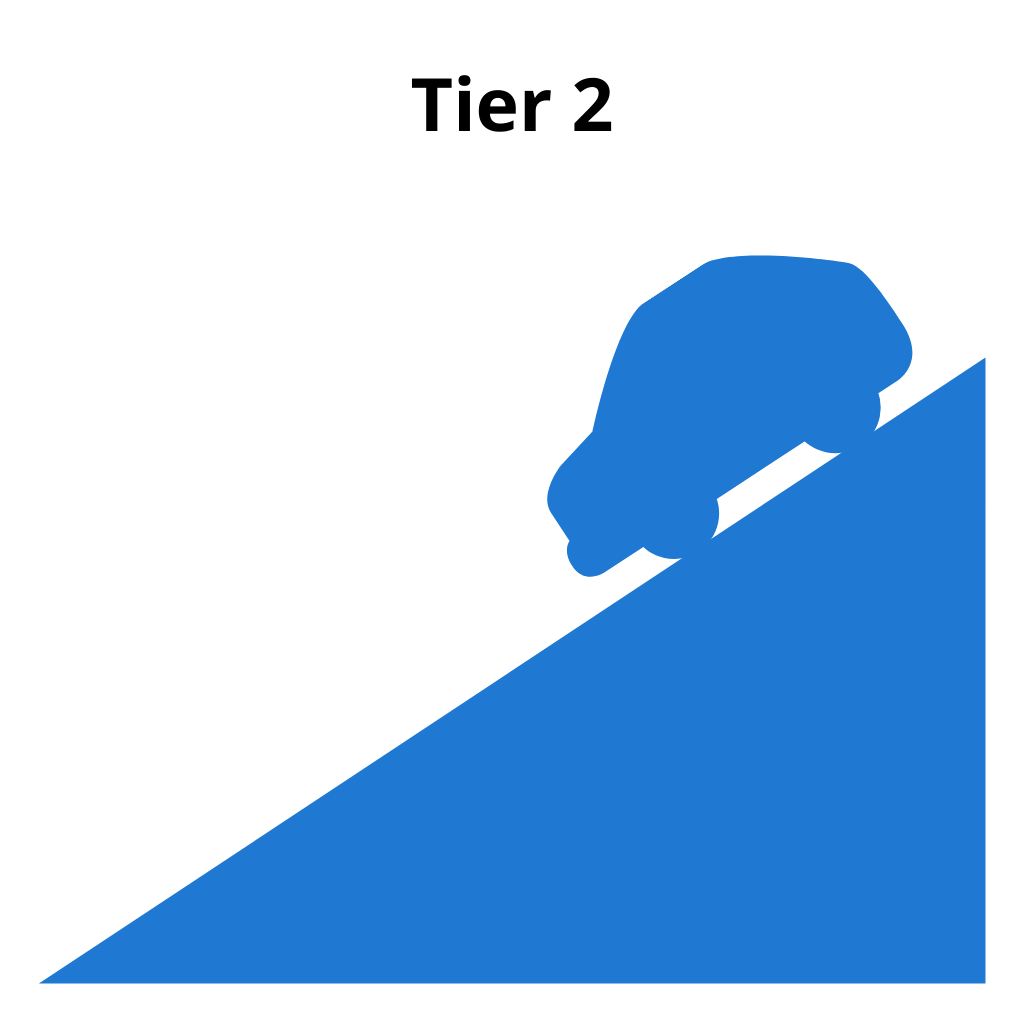In the lab students learn how to use kinematics equations to determine the acceleration of a mass along an inclined plane.

In the video the lab is performed followed by the data being presented on a graph, which students use to complete a lab sheet. When the graph is displayed, all the data needed to complete the sheet is presented both visually and verbally, including the values and units needed for calculations. What the information represents is also explained to the student.

## Videos - Lab 1 Inclined Plane Kinematics - Lab 1: Tier 3In the lab students learn how to use kinematics equations to determine the acceleration of a mass along an inclined plane.

In the video the lab is performed followed by the data being presented on a graph, which students use to complete a lab sheet. When the graph is displayed, all the data needed to complete the sheet is presented both visually and verbally, including the values and units needed for calculations. What the information represents is also explained to the student.

## Videos - Lab 2 Level PulleyIn the lab a cart is pulled across a level track by a hanging mass. Students determine the acceleration from a velocity vs. time graph and compare this with the acceleration that can be found using dynamics.

## Videos - Lab 2 Level Pulley - Lab 2: Tier 1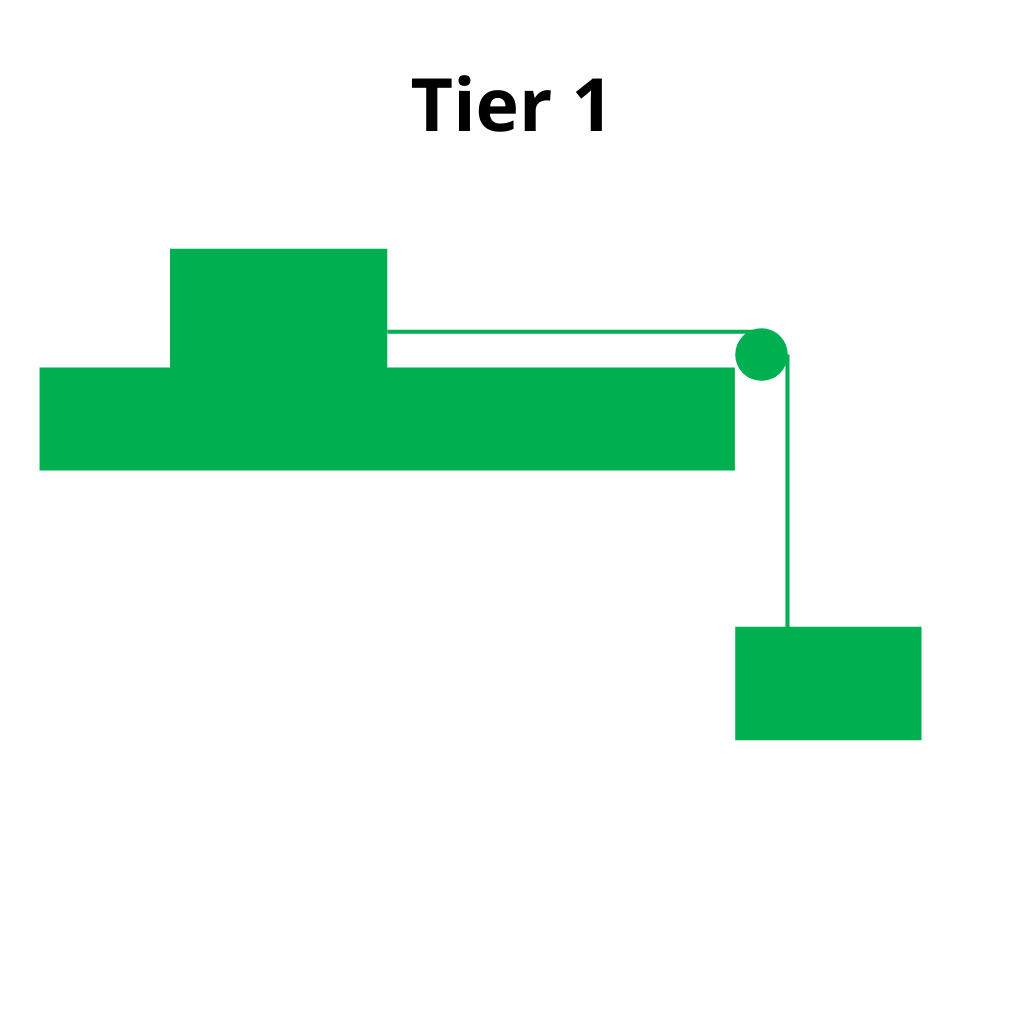In the lab a cart is pulled across a level track by a hanging mass. Students determine the acceleration from a velocity vs. time graph and compare this with the acceleration that can be found using dynamics.

In the video the lab is performed followed by the data being presented on a graph, which students use to complete a lab sheet. When the graph is displayed, all the data needed to complete the sheet is presented both visually and verbally, including the values and units needed for calculations. What the information represents is also explained to the student.

## Videos - Lab 2 Level Pulley - Lab 2: Tier 2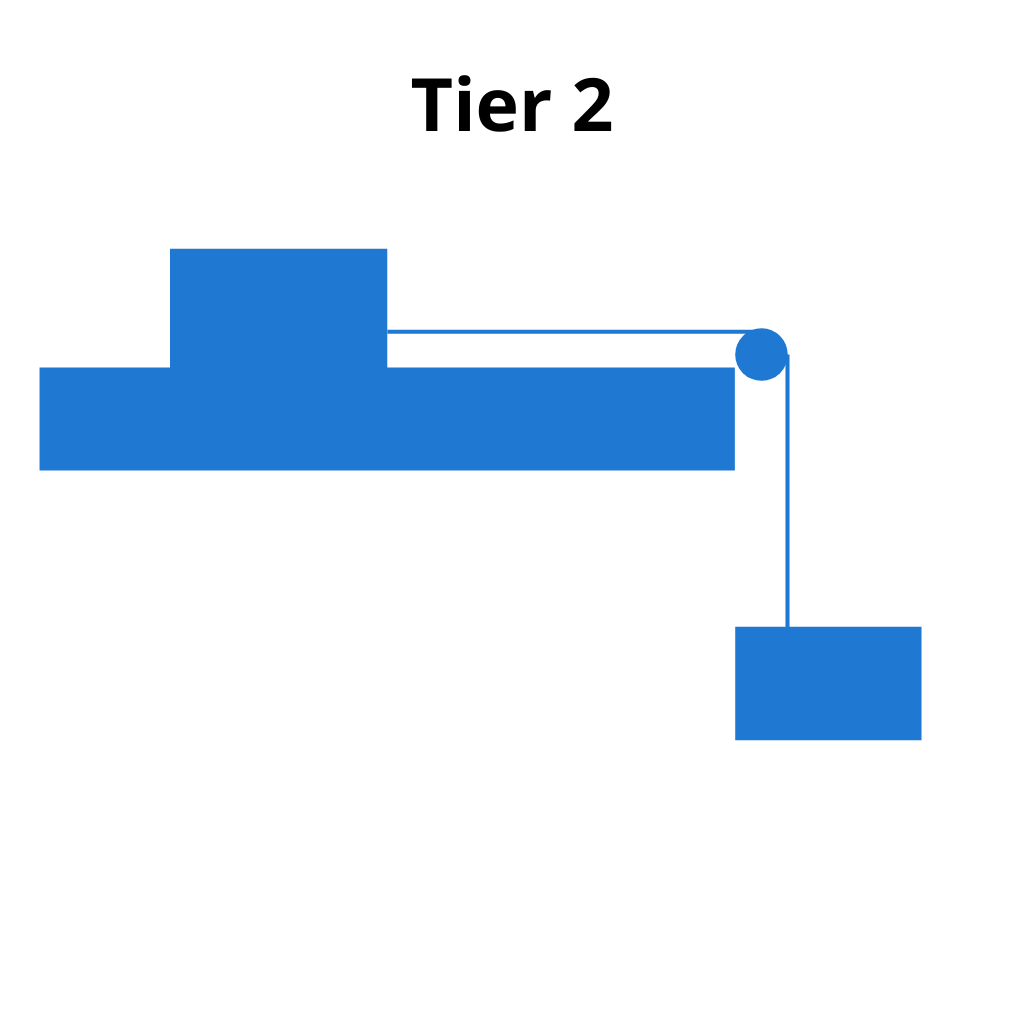In the lab a cart is pulled across a level track by a hanging mass. Students determine the acceleration from a velocity vs. time graph and compare this with the acceleration that can be found using dynamics.

In the video the lab is performed followed by the data being presented on a graph, which students use to complete a lab sheet. When the graph is displayed, all the data needed to complete the sheet is presented visually without a verbal description of what the data represents.

## Videos - Lab 2 Level Pulley - Lab 2: Tier 3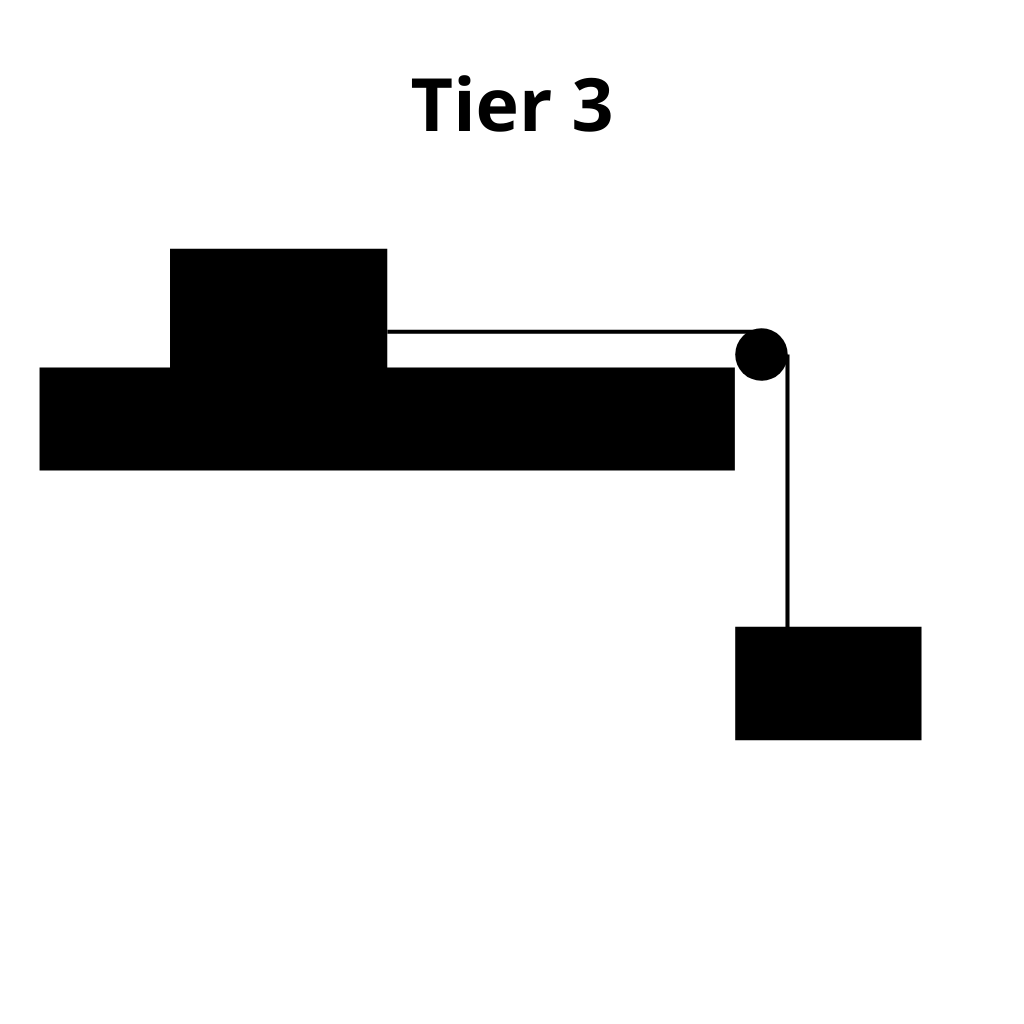In the lab a cart is pulled across a level track by a hanging mass. Students determine the acceleration from a velocity vs. time graph and compare this with the acceleration that can be found using dynamics.

In the video the lab is performed. The student must then load the data file into the graph to extract the necessary information to complete the lab sheet.

## Videos - Lab 3 Conservation of MomentumTwo carts of unequal mass are sent into a collision. The velocities of each cart, both before and after the collision, are found so that the total momentum before and after the collision can be determined and compared.

## Videos - Lab 3 Conservation of Momentum - Lab 3: Tier 1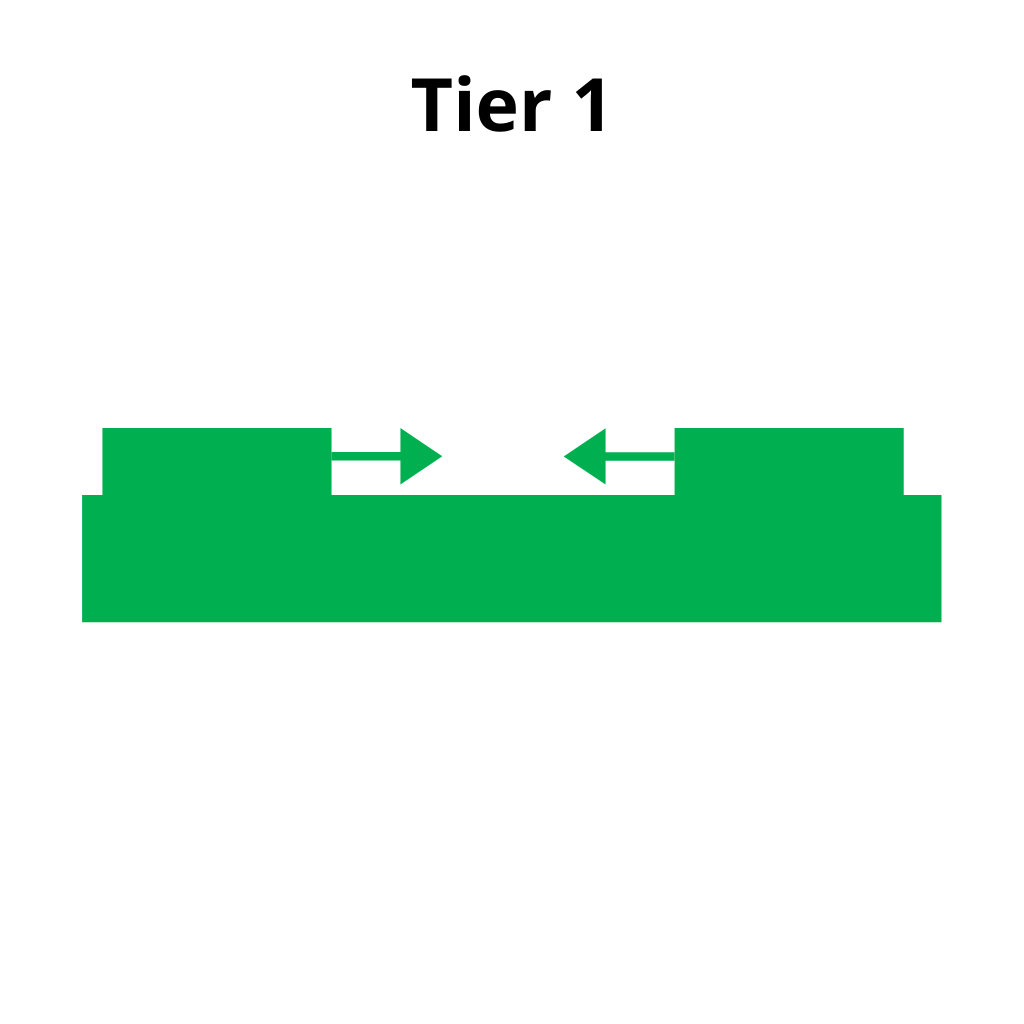Two carts of unequal mass are sent into a collision. The velocities of each cart, both before and after the collision, are found so that the total momentum before and after the collision can be determined and compared.

In the video the lab is performed followed by the data being presented on a graph, which students use to complete a lab sheet. When the graph is displayed, all the data needed to complete the sheet is presented both visually and verbally, including the values and units needed for calculations. What the information represents is also explained to the student.

## Videos - Lab 3 Conservation of Momentum - Lab 3: Tier 2Two carts of unequal mass are sent into a collision. The velocities of each cart, both before and after the collision, are found so that the total momentum before and after the collision can be determined and compared.

In the video the lab is performed followed by the data being presented on a graph, which students use to complete a lab sheet. When the graph is displayed, all the data needed to complete the sheet is presented visually without a verbal description of what the data represents.

## Videos - Lab 3 Conservation of Momentum - Lab 3: Tier 3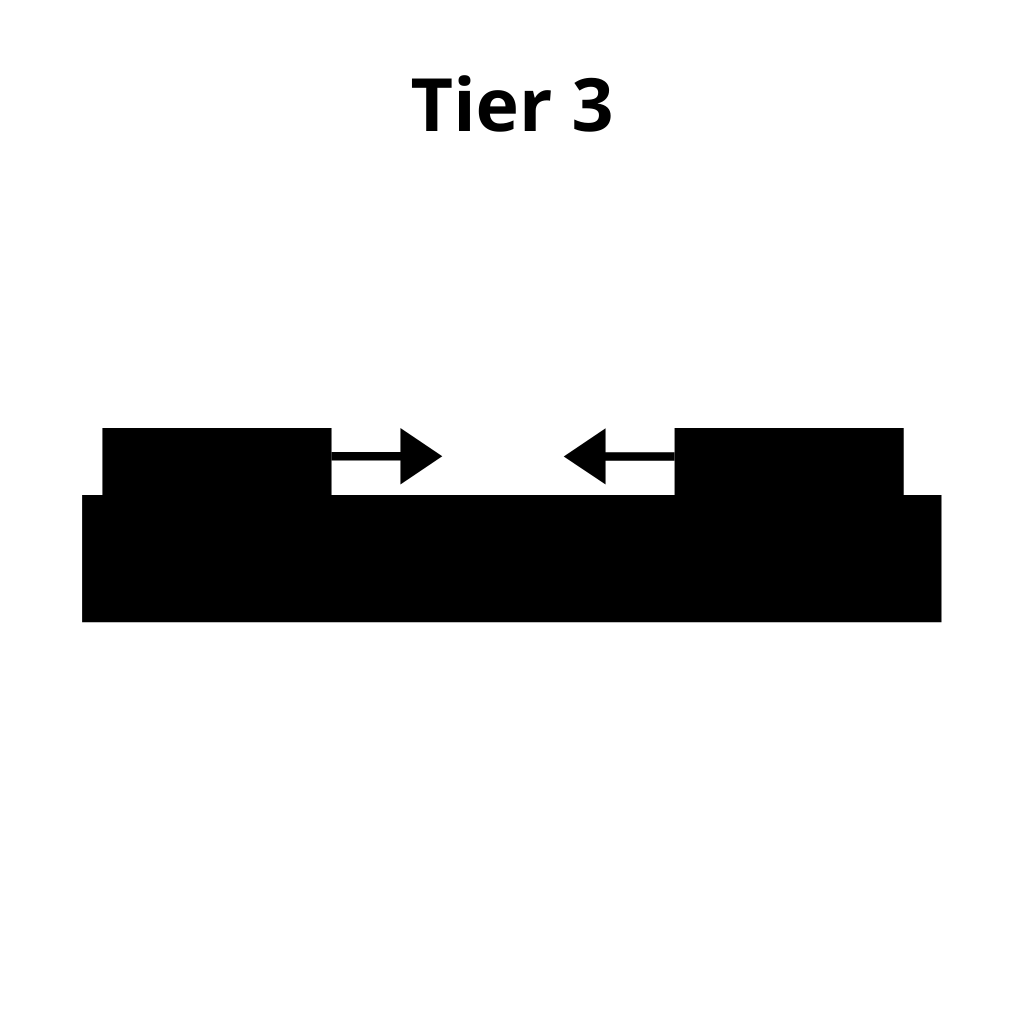Two carts of unequal mass are sent into a collision. The velocities of each cart, both before and after the collision, are found so that the total momentum before and after the collision can be determined and compared.

In the video the lab is performed. The student must then load the data file into the graph to extract the necessary information to complete the lab sheet.

## Videos - Lab 4 Inclined Plane EnergyA cart is released from rest at the top of an incline. The maximum velocity attained by the cart is found from a velocity vs. time graph to be compared with the velocity that can be found using energy.

## Videos - Lab 4 Inclined Plane Energy - Lab 4: Tier 1A cart is released from rest at the top of an incline. The maximum velocity attained by the cart is found from a velocity vs. time graph to be compared with the velocity that can be found using energy.

In the video the lab is performed followed by the data being presented on a graph, which students use to complete a lab sheet. When the graph is displayed, all the data needed to complete the sheet is presented both visually and verbally, including the values and units needed for calculations. What the information represents is also explained to the student.

## Videos - Lab 4 Inclined Plane Energy - Lab 4: Tier 2A cart is released from rest at the top of an incline. The maximum velocity attained by the cart is found from a velocity vs. time graph to be compared with the velocity that can be found using energy.

In the video the lab is performed followed by the data being presented on a graph, which students use to complete a lab sheet. When the graph is displayed, all the data needed to complete the sheet is presented visually without a verbal description of what the data represents.

## Videos - Lab 4 Inclined Plane Energy - Lab 4: Tier 3A cart is released from rest at the top of an incline. The maximum velocity attained by the cart is found from a velocity vs. time graph to be compared with the velocity that can be found using energy.

In the video the lab is performed. The student must then load the data file into the graph to extract the necessary information to complete the lab sheet.

## Videos - Lab 5 Rotational Dynamics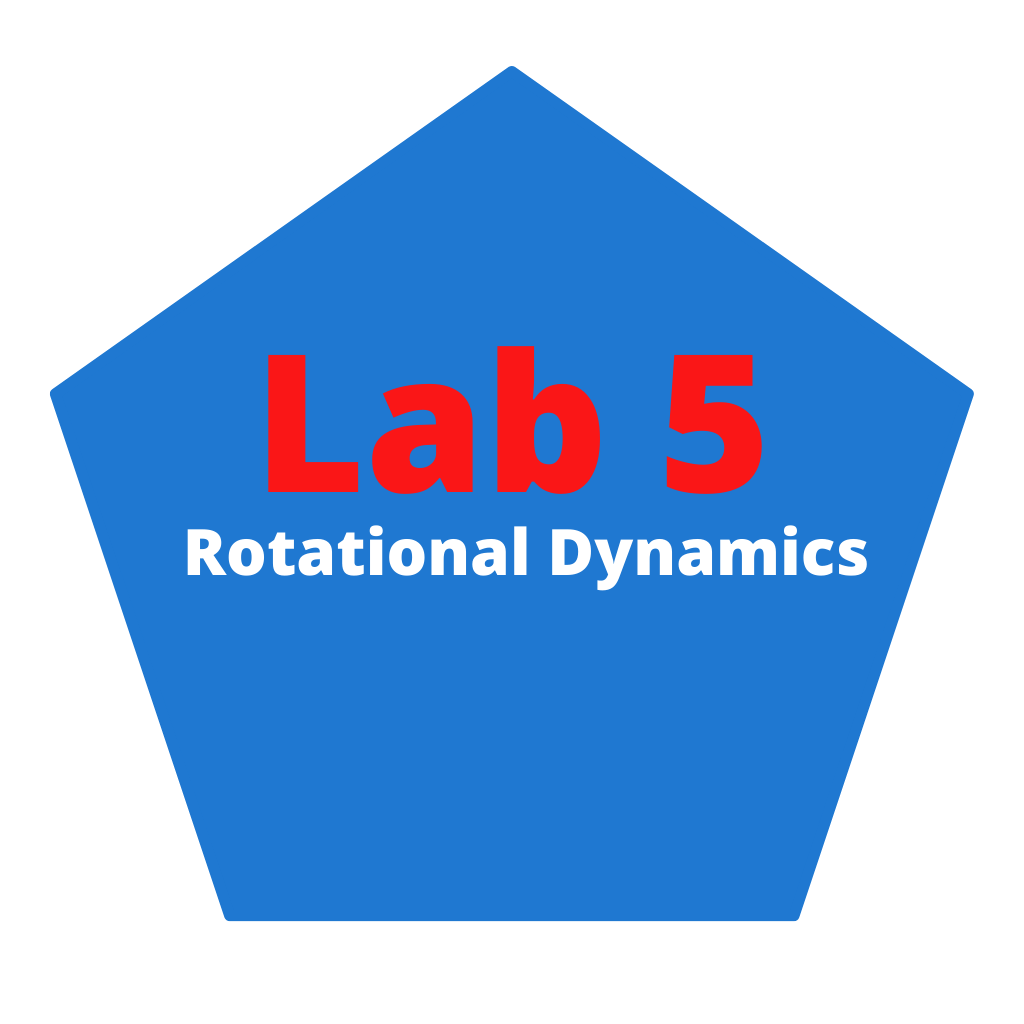A string is wrapped around a wheel and axle, draped over a pulley and attached to a hanging mass. When the mass is released it accelerates down. This acceleration is found. The calculations for this lab are intensive. There are conversions from inches to cm and a system of equations is solved using substitution.

## Videos - Lab 5 Rotational Dynamics - Lab 5: Tier 1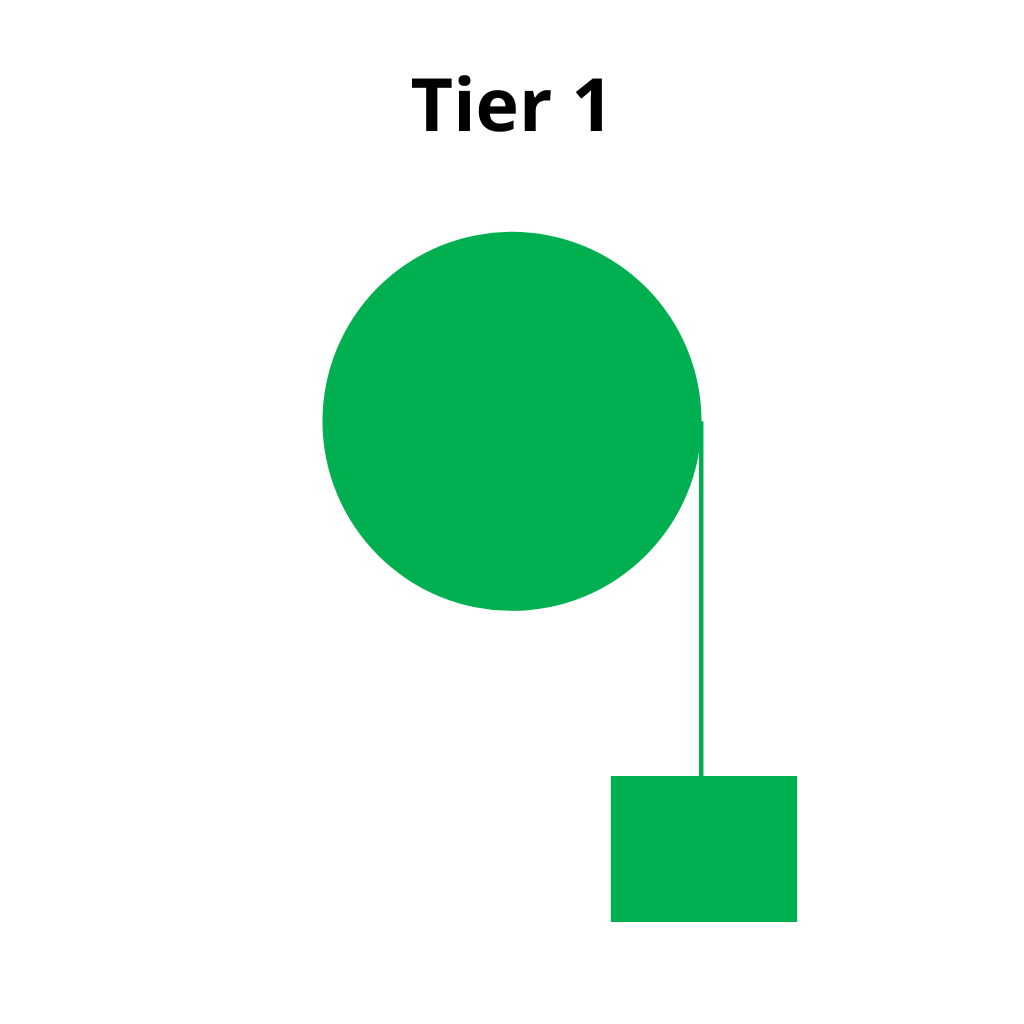A string is wrapped around a wheel and axle, draped over a pulley and attached to a hanging mass. When the mass is released it accelerates down. This acceleration is found. The calculations for this lab are intensive. There are conversions from inches to cm and a system of equations is solved using substitution.

In the video the lab is performed followed by the data being presented on a graph, which students use to complete a lab sheet. When the graph is displayed, all the data needed to complete the sheet is presented both visually and verbally, including the values and units needed for calculations. What the information represents is also explained to the student.